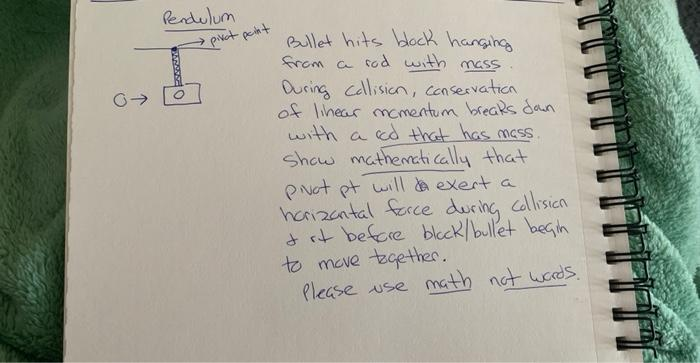# (Solved): in the ballistic pendulum, the blocj hangs from massless rod. we can then use conservation of linea ...in the ballistic pendulum, the blocj hangs from massless rod. we can then use conservation of linear momentum. however, when this rod now has mass. conservation of momentum breaks down. this is b/c the pivot point will exert a force on the rod. show this is the case by using physics formulas.
Bullet hits block hanging from a rod with mass During collisian, conservation of linear mamentum brears dan with a cod that has mass. show mathematically that pinot pt will exert a horizantal force during collision of it before black/bullet begin to mave trgether. please use math not words.

We have an Answer from Expert